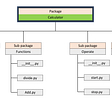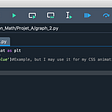# I. Elementary classes and objects

In Python, you can write code quickly and compile and run it pretty much on the spot. The compilation creates a byte code file and the Python runtime executes those byte codes pretty quickly. Python does not enforce a lot of type rules, because types are not resolved until execution time: any variable could change types any time you want.

Python doesn’t require semicolons or braces to define the end of statements and the boundaries of blocks of code. Instead, every statement is in a new line, and blocks of code are arranged by indenting all the statements.

But in some cases, Python runs pretty slowly compared to C++ and while Python is an object-oriented language, there are some things it just can’t do very well. So there are times when you wonder if coding in C++ would be better for your project.

It might be the case, but the C++ learning curve is considerably longer than that in Python, where you can begin writing code the same day you start to learn the language.

# Our Python Example

Let’s take a really simple class in Python represented a rectangle.

`# The Rectangle base class class Rectangle:def __init__(self, width, height):   self.width = width # save the dimensions   self.height = heightdef area(self): # compute the area   return self.height * self.width`

The initialization method (effectively the constructor) copies the width and height of the rectangle into the instance variables width and height for that class. The area method returns the area of the rectangle by multiplying the stored width and height.

Now let’s consider a class representing a Square, which is, of course, a kind of Rectangle where the sides are all the same length.

`# a Square is derived from Rectangle and has only one dimension class Square(Rectangle):    def __init__(self, side):       super().__init__( side, side) #pass both dims to parent class`

We derive this class from the Rectangle class, and in the initializer, we simple pass the side length up to the Rectangle base class twice: once as width and once as height.

# Getting the areas

So, if we want the areas of the Rectangle and Square, we only need write:

` rect= Rectangle(5, 4) print(“Rectangle,”, rect.area())  sq = Square(6) print(“Square,”, sq.area())`

And how is the area of the square calculated? There is no area method specified in the Square class, but there is one in the parent Rectangle class, and that’s the one that gets called. In the parent Rectangle class, the height and width were stored by the Square’s initializer method, and they are multiplied together and returned to the calling program right from the instance of the Square class. You don’t even have to mention it in the Square class! This is called inheritance.

The program prints out

`Rectangle, 20Square, 36`

If you know how to calculate the area of a circle, you can halve that for a semicircle. And the radius is either the width or height, which will have the same value.

`# Semicircle is derived from Square and needs only the radius class SemiCircle(Square):     def __init__(self, radius):         super().__init__( radius)      # area computation is different in this derived class     def area(self):         return (math.pi * self.width * self.width)/2`

We derive the Semicircle class from the Square class, since it has only one dimension: the radius. But in this class we override the base area method with a derived method that computes the area of a semicircle with that radius. This is sometimes called polymorphism, since you change the shape of the area method in the derived class.

Then, our complete program is

`rect= Rectangle(5, 4) print(“Rectangle,”, rect.area())  sq = Square(6) print(“Square,”, sq.area())  semi = SemiCircle(3) print(f’Semicircle, {semi.area():5.2f} ‘)`

and the resulting output is

`Rectangle, 20Square, 36Semicircle, 14.14`

# Rectangle classes in C++

In C++, you have to declare the type of every variable where you first use it. You can use white space and indentation any way you like: blocks of code are set up inside braces “{}.” So a very simple C++ statement might be

`int side = 12;`

You can also write that same statement as:

`int side {12};`

While these seem to do the same thing, there is one significant difference if you mix data types. If you write:

`float x = 6.02;int avo = x;`

there would be no error, because the C++ compiler automatically casts x to an integer before storing it in avo.

`float x = 6.02; int avo {x};`

the compiler will issue an error message telling you that you have perhaps unintentionally down-converted a float to an integer.

Code in C++ classes is always enclosed braces, and instance variables are declared within them. Unlike Python, these variables can be private, protected or public. Private variables can only be accessed by code within the class, protected variables can also be accessed by derived classes, and the less use public variables can be accessed by any code inside or outside that class.

Our basic Rectangle class, then, starts out like this:

`class Rectangle {// The dimensions can be accessed by child classesprotected:    float width, height;};`

Note that a class is terminated by a brace, followed by a semicolon.

Now, when you create an instance of the Rectangle class you must pass in the width and height values like this:

`Rectangle rect = Rectangle(5, 4);`

That way looks like Python, but a more compact C++ style does the same thing:

`Rectangle rect(5, 4);`

# The constructor

The constructor for any class is just a method having the class name and the parameters as arguments. So, for the Rectangle class, the constructor could be:

`Rectangle(float w, float h) {    width = w;    height = h;}`

But you can write this same constructor more succinctly using that brace notation we discussed above:

`//copy arguments into width and heightRectangle(float w, float h) : width{ w }, height{ h } {}`

Note that the values are copied into the instance variable after the colon and before the opening brace. This is far more succinct and once you get used to it, it’s easier to read. So our entire Rectangle class amounts to:

`//Base Rectangle classclass Rectangle {// The dimensions can be accessed by child classesprotected:    float width, height;public://copy arguments into width and height    Rectangle(float w, float h) : width{ w }, height{ h } {    }    float area() {        return height * width;    }};`

The positions of the braces are entirely up to you. You can also write the code with each brace on a new line:

`//Base Rectangle classclass Rectangle{// The dimensions can be accessed by child classes    protected:        float width, height;    public:        //copy arguments into width and height        Rectangle(float w, float h) : width{ w }, height{ h }            {                                                              }        float area()            {                 return height * width;            } };`

Indentation is also completely up to you, but some indentation makes classes much more readable, much as they are in Python.

# Derived classes

Now, suppose we want to make a Square class as we did in Python. It’s just about as simple, because you don’t even have to write an area method: it just calls the one in the parent class.

`// Derived Square class uses area method in parent classclass Square : public Rectangle {    public:        Square(float w) : Rectangle(w, w) {}};`

This is the whole class. Note that following the colon, you name the class it is derived from, and there you pass the Square width into the Rectangle parent class twice, representing the width and height (which are both the same).

We can also create a Semicircle object in just the same fashion. Now the area of a circle is πr².

So, the area of a semicircle is half that.

The only question is where do we get the value of pi in C++. You find it on the numbers library, and you would refer to it as

`std::numbers::pi`

A simpler way to write it is to invoke the using directive

`#include <numbers>using std::numbers::pi;`

so you can write pi directly as you see in our simple Semicircle class below:

`// Derived Semicircle overrides area methodclass Semicircle : public Square {    public:        Semicircle(float radius):Square(radius) {}        float area() {            return (pi * width * width) / 2;        }};`

Note that this is almost as simple as the square class. We just have to override the area method to compute the area using the equation we wrote just above.

# Creating class instances

In C++, every program starts in a function called main() and we create our class instances there. To print out the values of the area, we use the cout method, (pronounced “see-out”) and use a pair of less-than signs to point the values to the cout method.

Here, we create instances of the three classes and print out the areas:

`int main() {    Rectangle rect(5, 4);    cout << “Rectangle: “ << rect.area() << endl;    Square sq(6);    cout << “Square: “ << sq.area() << endl;    Semicircle semi(3);    cout << format(“Semicircle: {:3.2f}”, semi.area());}`

The output of this little program is

`Rectangle: 20Square: 36Semicircle: 14.14`

Note the C++ has a format method that is kind of like the Python f-string, although not quite as versatile. We’ll take this up in a later article.

These examples were developed and tested using Visual Studio 2022, Community Edition with the compiler set to use C++ 20 and “features from the latest working draft,” which includes the format statement.

The code for these examples can be found in GitHub under jameswcooper/articles/cppclasses as

· Rectangles.py, and

· Rectangles.cpp

--

--

--

is the author of “Python Programming with Design Patterns” and 20 previous books.

Love podcasts or audiobooks? Learn on the go with our new app.

## Design Pattern 101: Singleton and Factory Pattern## How to learn Matlab## Using Firebase Cloud Storage with Unity to share user generated content## Best Git Practices For Managing Your Project## FluentU Vs Drops: Which Is The #1 Best App?## Programming experience## How Kanban is Driving Agile Project Management## Selenium Element Interactions: Wait Strategies## James Cooper

is the author of “Python Programming with Design Patterns” and 20 previous books.

## Intro to Python ast Module## What are python packages and how to fix a python packages error “TypeError: ‘module’ object is not…## Pyplot: An introduction to the pyplot interface.## SUMIFS AND COUNTIFS in Python